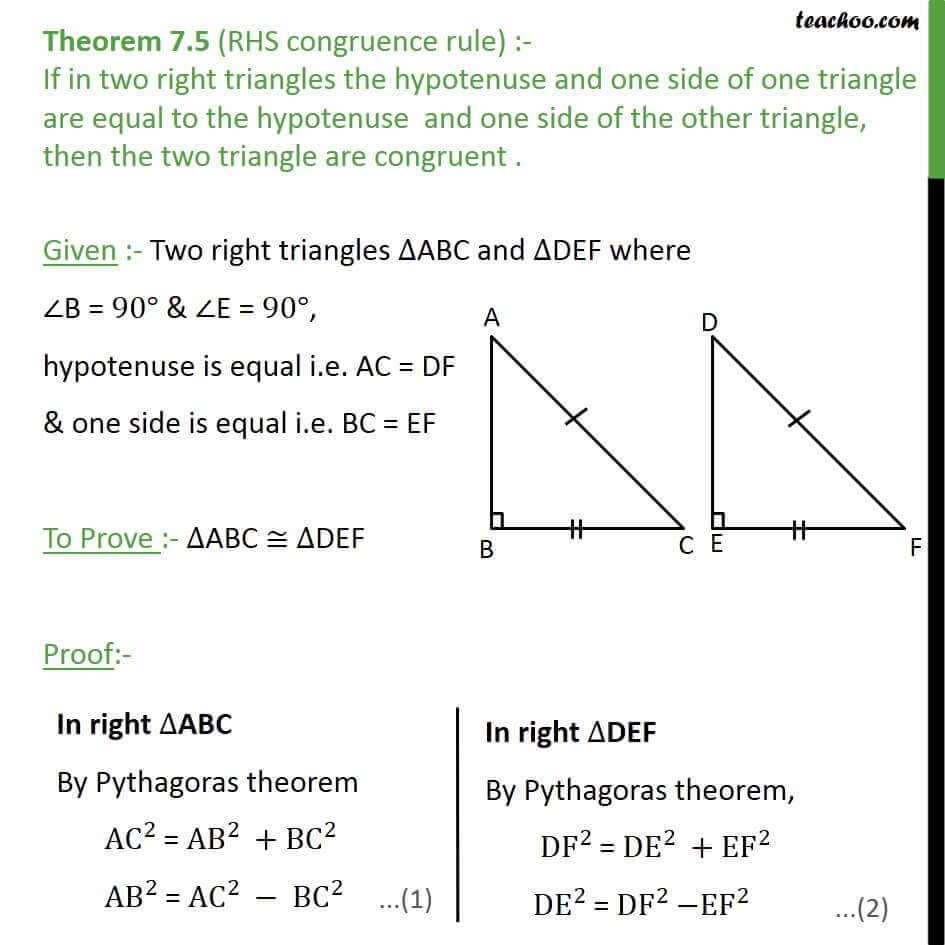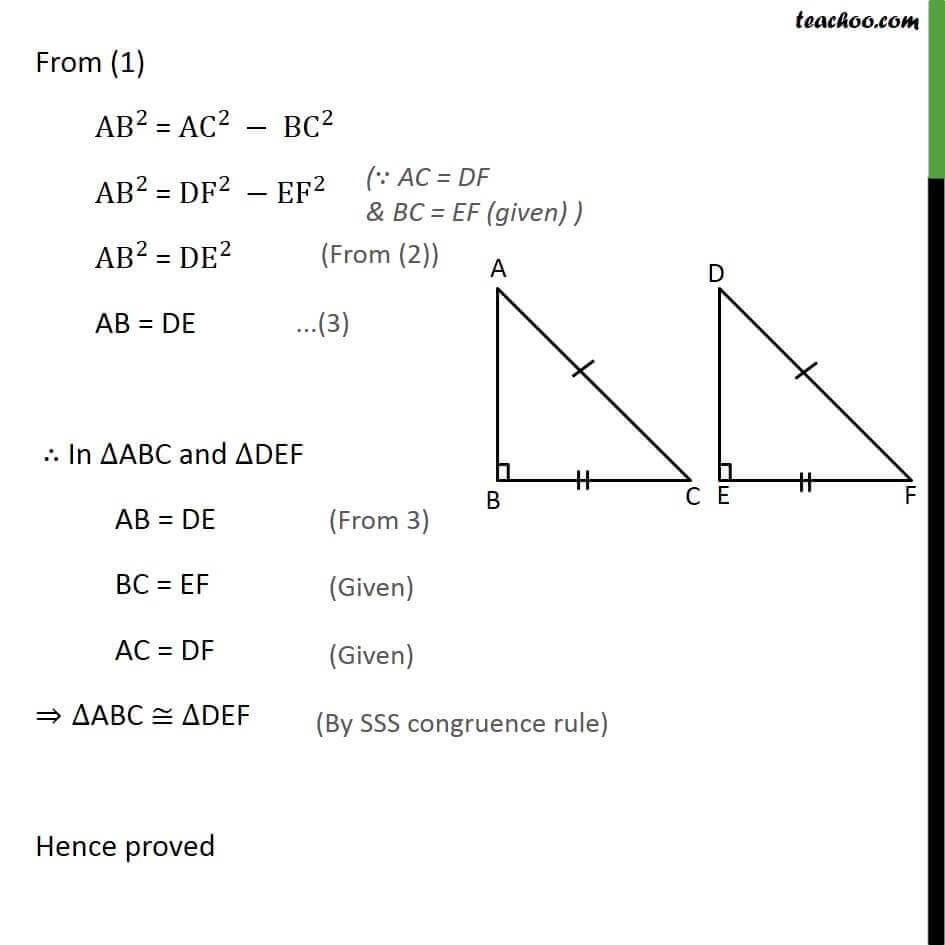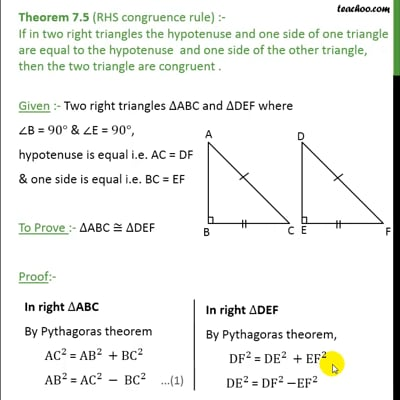RHS

Chapter 7 Class 9 Triangles
Concept wiseThis video is only available for Teachoo black users

Introducing your new favourite teacher - Teachoo Black, at only ₹83 per month

### Transcript

Theorem 7.5 (RHS congruence rule) :- If in two right triangles the hypotenuse and one side of one triangle are equal to the hypotenuse and one side of the other triangle, then the two triangle are congruent . Given :- Two right triangles ∆ABC and ∆DEF where ∠B = 90° & ∠E = 90°, hypotenuse is equal i.e. AC = DF & one side is equal i.e. BC = EF To Prove :- ∆ABC ≅ ∆DEF Proof:- From (1) AB﷮2 ﷯= AC﷮2 ﷯− BC﷮2 ﷯ AB﷮2 ﷯= DF﷮2 ﷯− EF﷮2 ﷯ AB﷮2 ﷯= DE﷮2 ﷯ AB = DE ∴ In ∆ABC and ∆DEF AB = DE BC = EF AC = DF ⇒∆ABC ≅ ∆DEF Hence proved